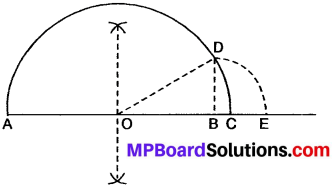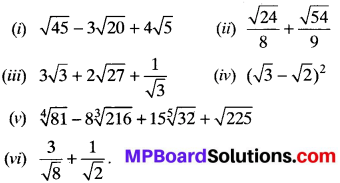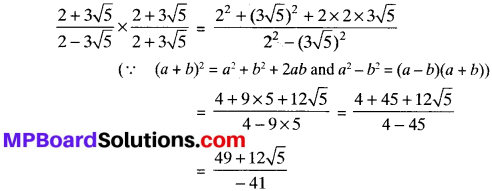# MP Board Class 9th Maths Solutions Chapter 1 Number Systems Ex 1.2

## MP Board Class 9th Maths Solutions Chapter 1 Number Systems Ex 1.2

Question 1.
State whether the following statements are true or false. Justify your answers.

1. Every irrational number is a real number.
2. Every point on the number line is of the form √m , where m is a natural number.
3. Every real number is an irrational number.

Solutions:

1. True, because all irrational numbers come in the collection of real numbers.
2. False, because a negative number cannot be the square root of any natural number.
3. False, because the collection of real numbers contains not only irrational numbers but also rational numbers.

Question 2.
Are the square roots of all positive integers irrational? If not, give an example of the square root of a number that is a rational number.
Solution:
No. For example, √4 = 2 is a rational number.

Question 3.
Show how √5 can be represented on the number line.
Solution:
Representing √5 on a number line as shown in fig. below.1. Take OA = 2 units and draw perpendicular AB on A.
2. Cut AB = 1 unit and join OB.
3. By taking O as centre and OB as radius, draw an arc which inter-sect the number line at C.

Hence OC = √5
∴ Point C represents ^5 on the number line.
Proof:
OBD is a right angled A.
∴ OD2 = OB2 + BD2
OD2 = (2)2 + (1)2 = 5
OD = √5Representation of Real Numbers on Number Line

Real Numbers:
The rational and irrational numbers taken together are known as real numbers. Every real number Is either rational or irrational. Different operations on real numbers are shown below:
For example:Example 1.
Add (3√2 + 5√3) and (√2 + √3).
Solution:
(3√2+ 5√5)+ (√2+ √3)
= 3√2 + 5√5 + √5 + √5 = (3√5+ √5)+ (5√5+ √5)
= 4√2 + 6√3
= 4√5 + 6√5

Example 2.
Add (√5 + 7√5) and (4√3 + 6√5).
Solution:
(√5 + 7√5) + (4√3 + 6√5)
= √3 + 7√5 + 4√3 + 6√5
= (√3 + 4√3) + (7√5 + 6√5)
= 5√3 + 13√5.

Example 3.
Simplify (3 + √2) (3 – √2).
Solution:
(3)2 – (√2)2
(∴ a2 – b2 = (a + b) (a – b))
= 9 – 2
= 7.Example 4.
Divide 16√6 by 4√2 .
Solution:
16√6 ÷ 4√2
$$\frac { 16\sqrt { 6 } }{ 4\sqrt { 2 } }$$
4√3.

Example 5.
Simplify the following:Solution:
(i) $$\sqrt{45}$$ – 3$$\sqrt{20}$$ = 4$$\sqrt{5}$$ = $$\sqrt{9×5}$$ – 3$$\sqrt{4×5}$$ + 4$$\sqrt{5}$$Example 6.
Simplify by rationalising the denominator of $$\frac { 2+3\sqrt { 5 } }{ 2-3\sqrt { 5 } }$$
Solution:
We have 3 $$\frac { 2+3\sqrt { 5 } }{ 2-3\sqrt { 5 } }$$, multiply numerator and denominator by 2 + 3√5, we have